Scalable Kernel Learning and Gaussian Processes

Update: KISS-GP and Deep Kernel Learning code has just been released! (Oct 24, 2016)
Update: GP-LSTM paper and code has just been released! (Oct 28, 2016)
Update: Expanded the README in the Deep Kernel Learning source (Oct 28, 2016)
Update: The SV-DKL paper and code have been posted! (Nov 2, 2016)

Kernel methods, such as Gaussian processes, have had an exceptionally consequential impact on machine learning theory and practice.  However, these methods face two fundamental open questions:

(1) Kernel Selection:  The generalisation properties of a kernel method entirely depend on a kernel function.  However, often one  defaults to the RBF kernel, which can only discover very limited representations of data.

(2) Scalability:  Gaussian processes, for example, scale as O(n^3) for training, O(n^2) for storage, and O(n^2) per test prediction,
for n training points.  This scalability typically limits GP applicability to at most a few thousand datapoints.  Moreover, standard approaches to GP scalability typically only work for up to about fifty thousand training points, and sacrifice too much model structure for kernel learning.

We view kernel learning and scalability as two sides of one coin.  We most want flexible models on large datasets, which often contain the necessary information to learn rich statistical representations through kernel learning -- for great predictive performance, and new scientific insights into our modelling problems.

There has been exciting recent work targeted at addressing these limitations.

On this page, we have code, tutorials, demos, and resources for our work on scalable kernel learning.   We introduce approaches for scalable inference and automatically learning kernel functions.  Several of these approaches have the inductive biases of deep learning architectures, while retaining a probabilistic non-parametric representation, and allowing for O(n) training and O(1) testing (compared
to O(n^3) and O(n^2) for standard GPs).  Our approaches to scalability are largely grounded in exploiting algebraic structure in kernel
matrices in combination with local kernel interpolation, for efficient and highly accurate numerical linear algebra operations.

Moreover, we view kernel methods and deep learning as complementary rather than competing approaches.  Kernel methods provide an elegant mathematical approach to representing an infinite number of basis functions using a finite amount of computional power.   Deep learning approaches provide a way to create adaptive basis functions which have powerful inductive biases.  With deep kernel learning, we can create infinite expansions with basis functions that have the structural properties and inductive biases of neural networks.  The information capacity of such models grows automatically with the size of the data, and the complexity can be automatically calibrated through Bayesian inference.  And by viewing neural networks through the lens of metric learning, deep learning approaches become more interpretable.

Many of these themes are described in my talk "Scalable Gaussian Processes for Scientific Discovery".

We always welcome constructive feedback.  Please feel free to reach out with bug reports or suggestions.  To understand this material
you must have a basic understanding of Gaussian processes as described in the first five chapters of GPML (particularly chapters 2, 4, 5), or in the first two chapters of my PhD thesis.

All of the code here builds upon the GPML library.  Before using this code, I suggest following some of the tutorials on the GPML website.

To jump navigate to the various sections:
[Spectral Mixture Kernels | Kronecker Inference | Kronecker non-Gaussian Likelihoods | KISS-GP | Deep Kernel Learning][Stochastic Variational Deep Kernel Learning | GP-LSTMDatasets]

Andrew Gordon Wilson
November 2, 2016

Spectral Mixture Kernels

The spectral mixture (SM) kernel is an expressive basis for all stationary covariance functions.  It is formed by modelling the spectral
density (Fourier transform) of a kernel as a scale-location Gaussian mixture.  One can analytically take the inverse Fourier transform to recover the closed form expression for this kernel.  Learning the kernel amounts to learning the hyperparameters of this kernel function, using the same techniques as one would learn the hyperparameters of an RBF kernel, but with special care for how these parameters are initialized.  This kernel has been used to discover a wide range of flexible statistical representations, enabling long range crime prediction, time series, image and video extrapolation, as well as new interpretable scientific insights into our modelling problems.

Key Reference:

Gaussian process kernels for pattern discovery and extrapolation
Andrew Gordon Wilson and Ryan Prescott Adams
International Conference on Machine Learning (ICML), 2013.
[arXiv, PDF, Supplementary, Slides, Video Lecture, Typo correction, BibTeX]

Typo correction regarding the spectral mixture kernel for multidimensional inputs

Our other papers discussing spectral mixture kernels:

Covariance kernels for fast automatic pattern discovery and extrapolation with Gaussian processes
Andrew Gordon Wilson
PhD Thesis, January 2014
[PDF, BibTeX]

Fast kernel learning for multidimensional pattern extrapolation
Andrew Gordon Wilson*, Elad Gilboa*, Arye Nehorai, and John P. Cunningham
Advances in Neural Information Processing Systems (NIPS) 2014
[PDF, BibTeX, Code, Slides]

Kernel Interpolation for Scalable Structured Gaussian Processes (KISS-GP)
Andrew Gordon Wilson and Hannes Nickisch
To appear at the International Conference on Machine Learning (ICML), 2015
[PDF, Supplement, arXiv, BibTeX, Theme Song]

Fast kronecker inference in Gaussian processes with non-Gaussian likelihoods
Seth Flaxman, Andrew Gordon Wilson, Daniel Neill, Hannes Nickisch, and Alexander J. Smola
To appear at the International Conference on Machine Learning (ICML), 2015
[PDF, Supplement, Code]

À la carte - learning fast kernels
Zichao Yang, Alexander J. Smola, Le Song, and Andrew Gordon Wilson
Artificial Intelligence and Statistics (AISTATS), 2015
Oral Presentation
[PDF, BibTeX]

A process over all stationary covariance kernels
Andrew Gordon Wilson
Technical Report, University of Cambridge.  June 2012.
[PDF]

Scalable Gaussian Processes for Characterizing Multidimensional Change Surfaces
William Herlands, Andrew Gordon Wilson, Seth Flaxman, Daniel Neill, Wilbert van Panhuis, and Eric P. Xing
Artificial Intelligence and Statistics (AISTATS), 2016
[PDF, BibTeX]

Deep Kernel Learning
Andrew Gordon Wilson*, Zhiting Hu*, Ruslan Salakhutdinov, and Eric P. Xing
Artificial Intelligence and Statistics (AISTATS), 2016
[PDF, arXiv, BibTeX]

The Human Kernel
Andrew Gordon Wilson, Christoph Dann, Christopher G. Lucas, and Eric P. Xing
Neural Information Processing Systems (NIPS), 2015
Spotlight
[PDF, arXiv, Supplement, BibTeX]

Code:
- GPML v3.6 [older version]
- covSM.m
- covSMfast.m [only works if the inputs are 1D]
- initSMhypers.m
- smspect.m
- empspect.m
- plotspectral.m
- testSMairline.m
- testSMCO2.m
- airline dataset
- CO2 dataset
- audio dataset
- everything for this demo (except GPML) in one zip

The spectral mixture kernel has a simple form: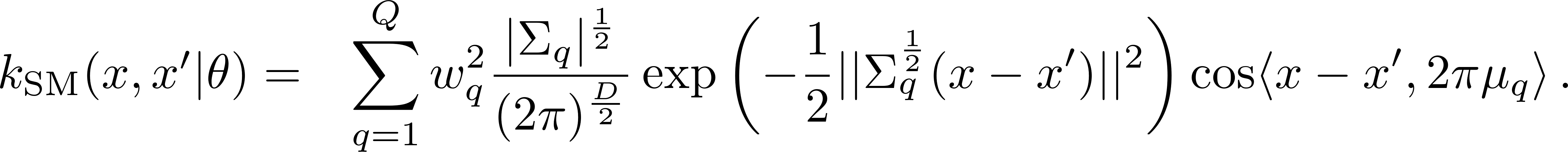Here theta contains all of the kernel hyperparameters, and Sigma_q in one dimension simply represents a variance parameter v_q.

In some papers (and code) the weights, w_q, are squared, in others they are not.  Just be careful to keep track of whether the weights are squared and adjust your interpretation accordingly.

One can learn the hyperparameters by maximizing the log marginal likelihood objective (with training data y):Or one can intergrate away these hyperparameters using sampling techniques.

Running a demo:

Step 2: Run testSMairline.m to produce the following time series extrapolation results
(I have included an example run result in airlinerun.mat):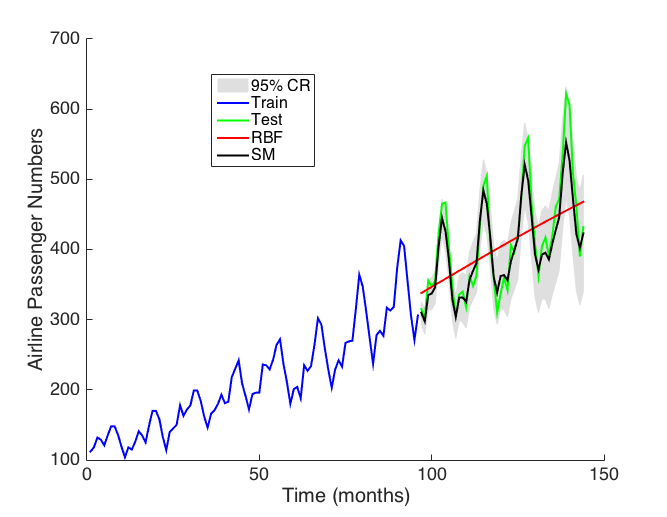Step 3: Try the same experiment with different random seeds.  Do you find consistent results?
Replace 'covSM.m' with 'covSMfast.m'.  How does it affect the runtime of testSMairline?
[The newer versions of covSM.m are fast and you may not notice a difference].

Understanding the parameters:
Step 4: The spectral mixture kernel is flexible, capable of discovering many powerful representations, but the learning surface for a spectral mixture kernel is often highly multimodal, making initialization very important for good predictive success.  Thankfully there are several initialization routines for the spectral mixture kernel which are reasonably simple and work on a variety of datasets with different properties.

There are four key hyperparameters: the weights (w_i^2), variances (v_i), and means (µ_i) of the Gaussians in frequency space, and the noise variance (sigma^2) in the time domain.

The variances can be thought of as inverse length-scales, and means as frequencies, and the weights as signal variances.

Step 5: Set the number of components Q = 1.  Hard-code the variance to zero, the frequency to 2, the weight to 1, and plot a draw from a GP with this spectral mixture kernel.  Notice that it's a strictly periodic function with a period of 0.5.  Now take a draw with the variance set to 1.  The resulting draws should become quasiperiodic.

Step 6: We can gain more insights into the parameters of the spectral mixture kernel by looking at its associated spectral density.  Plot the (log) spectral densities, with help from the smspect.m associated with these two models.  See what happens, both in function space, and in the spectral density, as you vary the frequency, weight, and variance (inverse length-scale) parameters to their extreme values, and add more components to the model.

Run plotspectral.m on the learned kernel for airline to reproduce this plot: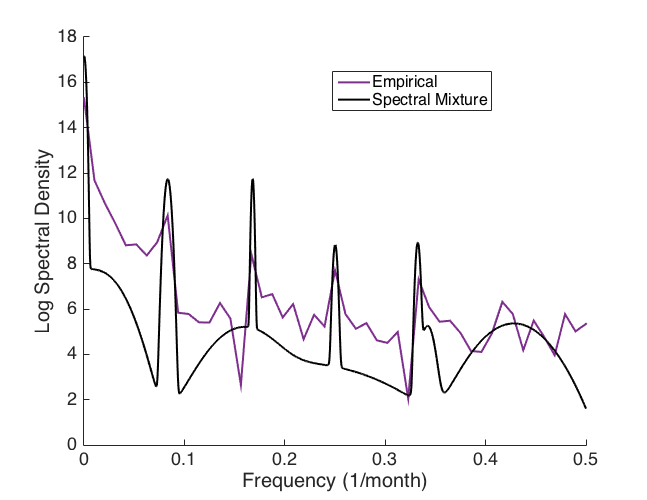Notice that the empirical spectrum looks like a very noisy version of the learned spectral mixture spectral density, with major frequency peaks in the same places [we will use this later for initialization].

Initialization:

Step 7: The SM kernel can match a wide range of different covariance functions corresponding to different settings of its hyperparameters.  However, learning a good set of hyperparameters can be difficult, because the learning objective is typically quite multimodal in the frequency parameters.  On the airline dataset, try looking at the log marginal likelihood as a function of the frequency parameters, fixing the variances and weights.

Step 8: In step 2 we used the script initSMhypers.m to initialize the spectral mixture kernel.  It's a relatively simple procedure, where we initialize the

weights: the weights are related to the signal variance of the data.  If we look at k(0,0) we get the sum of the weights.  This value is also the signal variance of our distribution over functions.  We therefore initialize the weights as the standard deviation of the data divided by the number of components (assuming that the noise standard deviation is negligable compared to the signal).  All the weights are initialized to the same value here.

variances: I find it helpful to think of the variances as inverse length-scales.  We take the range of the data (which is roughly an upper bound on the length-scale we might expect), and then sample from a truncated Gaussian distribution with a mean equal to the range to get these parameters.

frequencies: the hardest part.  I often think of the frequency parameters in terms of periods (1/frequency). The marginal likelihood will be most multimodal in these parameters. However, our approach is simple: we initialize using a uniform distribution from 0 to the
Nyquist frequency.  When the data are not regularly spaced, the "Nyquist frequency" typically doesn't exist.  However, in these cases we would not be likely to see a frequency bigger than Fs = 1/[smallest spacing between two points in the data per dimension], as there would be no justification for a period smaller than this spacing.  This parameter, Fs, turns out to be critical to finding a good initialization.

For any given problem, you should put some thought into a good setting for Fs, and try varying Fs to see how it affects the results.

We can then evaluate the marginal likelihood a number of times (e.g. 100) sampling from this initialization script each time, and start optimizing the marginal likelihood with the initialization that has the highest marginal likelihood [this is what we do in the next section on image inpainting].  Alternatively, we can try a smaller number (e.g. 10) of short optimization runs, and then a longer run, starting with the initialization that had the highest marginal likelihood after the short runs.  Try both approaches and see which you prefer!  In particular, in testSMairline.m, try playing with the trade-off between the parameters numinit and shortrunRemember to use a different random seed for each run! (The default is set to 42).  Try to find a better initialization by tuning the initialization routine to a setting which has more consistently good results across different random seeds.

Step 9:  In Step 8, we considered a simple initialization strategy.  It won't always work nicely, particularly if you can't find a good setting for Fs.  Take a look at initSMhypersadvanced.m.  Here we take a look at the empirical spectral density (see Chapter 2, page 62, of my thesis) which is proportional to the FFT of the squared data.  Recall in Step 6 we looked at the log empirical spectral density (compared to the successful learned spectral mixture kernel log spectral density) for the airline dataset, by running plotspectral.m
Notice that all of the major peaks in the empirical spectrum appear to be in the right places.  We can use this property to find a good initialization for the frequency parameters.

Step 10: The empirical spectral density is often too noisy to use to directly create a good kernel function. However, it often has its sharp peaks near the right frequencies.  We can treat the empirical spectral density as observed, and then directly fit a mixture of Gaussians to this empirical spectral density, using a standard Gaussian mixture density estimator (by taking samples from the empirical inverse CDF of the spectral density).  We can then use the fitted parameters as an initialization for the spectral mixture kernel.  We do this in the initSMhypersadvanced.m.  For variety, try running testSMCO2_adv.m. (try running a few times to see any differences in results).  I've included the output run shown here in CO2run.mat.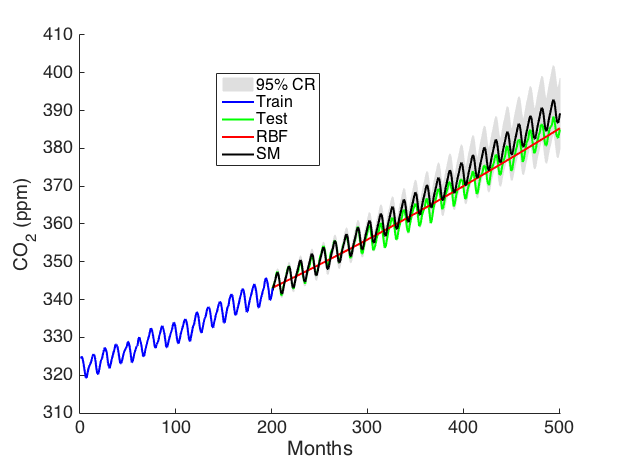Step 11: In addition to fitting the empirical spectral density, script testSMCO2_adv.m starts the initialization with a stochastic mini-batch approach (using gradient descent with momentum). The marginal likelihood of a GP does not factorize, so stochastic gradient descent will not be provably convergent.  However, SGD is a reasonable way to initialize the full batch optimization procedure for spectral mixture kernels.  The idea is that the bad local optima for frequencies will be in different places for different mini-batches, but the good global optima will be in similar places in each batch.

Step 12: Try the included audio dataset, and look at an initialization that works well for these data.  In general, try writing your own initialization scripts.  There are many ways to initialize the spectral mixture kernel, and I haven't found a magic bullet (but you might!).  There are some more advanced ideas in our work on change surfaces.  I have found initialization gets easier with more data.  If you are interested in using the SM kernel, I highly recommend following the next tutorial, which considers new initialization scripts for more data and multidimensional inputs.

Kronecker Inference with Spectral Mixture Kernels

When inputs are on a multidimensional grid (e.g., a Cartesian product across grid dimensions), and the kernel decomposes as a product across grid dimensions, then the data covariance matrix decomposes as a Kronecker product of kernels operating on each individual grid dimension.

Consider, for example, modelling a 1000 x 1000 pixel image, using a GP with an RBF kernel.  The inputs are pixel locations (in R^2), and the outputs are pixel intensities.  There are therefore 1 million datapoints, and the corresponding 10^6 x 10^6 covariance matrix could not normally even be represented on a computer.  However, in this case, the inputs are on a fully connected two dimensional grid: every horizontal pixel coordinate has an associated vertical pixel coordinate.  Moreover, the RBF (or, more generally, ARD) kernel decomposes as a product of RBF kernels acting on each input dimension.  This decomposition corresponds to a soft a priori assumption of independence across input dimensions, but it does not rule out posterior correlations, and in fact, provides a useful inductive bias.

Therefore the 10^6 x 10^6 covariance matrix will decompose into a a Kronecker product of two 10^3 x 10^3 covariance matrices, enabling exact inference and learning at the computational cost of working with about 10^3 datapoints!

In this section, we will demo a method which allows Kronecker inference even in the case of partial grid structure: suppose we have missing pixels in an image, or water covering up part of a map in a spatiotemporal statistics problem. Moreover, we will combine this scalable and exact inference with spectral mixture kernels, with demos on image inpainting problems.

The main reference for this section is:

Fast kernel learning for multidimensional pattern extrapolation
Andrew Gordon Wilson*, Elad Gilboa*, Arye Nehorai, and John P. Cunningham
Advances in Neural Information Processing Systems (NIPS), 2014
[PDF, BibTeX, Code, Slides]

Other references which use this methodology include:

Covariance kernels for fast automatic pattern discovery and extrapolation with Gaussian processes
Andrew Gordon Wilson
PhD Thesis, January 2014
[PDF, BibTeX]

Fast kronecker inference in Gaussian processes with non-Gaussian likelihoods
Seth Flaxman, Andrew Gordon Wilson, Daniel Neill, Hannes Nickisch, and Alexander J. Smola
International Conference on Machine Learning (ICML), 2015
[PDF, Supplement, Code]

Scalable Gaussian Processes for Characterizing Multidimensional Change Surfaces
William Herlands, Andrew Gordon Wilson, Seth Flaxman, Daniel Neill, Wilbert van Panhuis, and Eric P. Xing
Artificial Intelligence and Statistics (AISTATS), 2016
[PDF, BibTeX]

Video Resource:
Scalable Gaussian Processes for Scientific Discovery (EPFL, Februrary 2016)

Audio Resource:
Abstract, Slides, and Audio, for my November 2014 Oxford lecture "Kernel Methods for Large Scale Representation Learning"

2) For the tutorials, the only required external files are 'vincent.m', 'spectral_init.m', 'test_covGrid.m', 'test_infGrid.m', and the datasets.  'covSMfast.m' is an optional faster implementation of covSM.  All tutorial files are available in this zip file.   Unzip these tutorial files into one directory.

Example 1:   Try running 'test_covGrid.m' and 'test_infGrid.m' .  These show the accuracy of the grid methods compared to standard methods involving Cholesky decompositions.

covGrid is used to generate a covariance matrix with Kronecker product structure, which can be exploited by infGrid.   This test shows the generated covariance matrix is identical to what would be obtained using standard methods.

infGrid exploits Kronecker structure for scalable inference and learning.  In the case where the inputs are on a grid, with no missing inputs, the inference and learning is also exact.  In this demo, the data only has partial grid structure.  Every second data point is missing, as indicated by idx = (1:2:N)'.  The idx variable contains the locations of the training points. We use the extensions in Wilson et. al (2014) to handle partial grids efficiently.  With partial grids, inference requires linear conjugate gradients (LCG).  With a high tolerance (< 1e-4), and number of iterations (> 400), inference is exact to within numerical precision.  Typically, a tolerance of 1e-2 and maximum iterations of 200 is sufficient. With partial grids, the log determinant of the covariance matrix, used for marginal likelihood evaluations, undergoes a small approximation.

Exercise 1: Try adjusting opt.cg_maxit and opt.cg_tol, and observe the effect on inference.
Exercise 2: Try changing
idx to idx = (1:1:N)' and see what happens.
Exercise 3: Cycle between the three test cases, cs = 1, 2, 3.

Example 2: Try running 'vincent.m'.  You will be given a choice of patterns, and the code will extrapolate these patterns automatically.  This script reconstructs some of the results in
Fast Kernel Learning for Multidimensional Pattern Extrapolation.

Do not worry if there are occasional warnings that LCG has not converged.  The optimization will proceed normally.  The warnings can be resolved by adjusting the tolerance and maximum number of iterations.  We have set these values for a good balance of accuracy and efficiency, not minding if LCG occasionally does not converge to within the specified precision.

Some example output: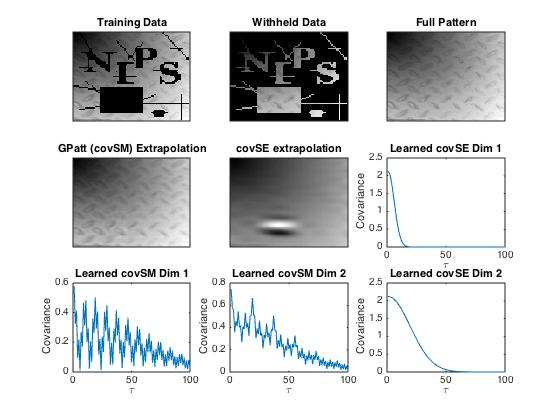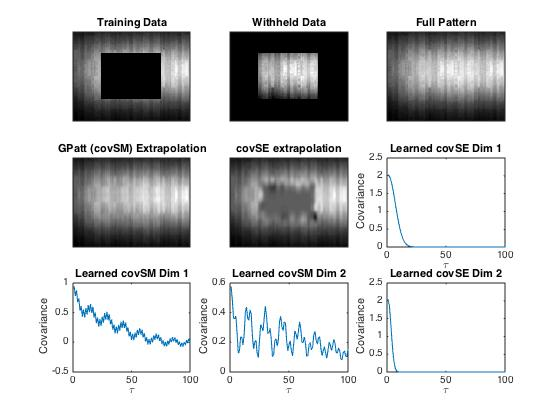Note the learned spectral mixture kernels.  It would be difficult to know to a priori hard code kernels like these.  The learned structure is interpretable and enables good predictions and extrapolation.

Exercise 1:  Extrapolations are produced with spectral mixture kernels and SE kernels.  Try adding a Matern kernel to this demo.  It will do better on the letters example than an SE kernel, but not as well as the spectral mixture kernel on larger missing regions.

Exercise 2:  Study the initialisation script spectral_init.m.   This is a basic and robust initialisation for spectral mixture kernels.  We sample initial parameters from several basic distributions multiple times, and then start the optimization run from the initialization with the highest marginal likelihood.  One of the critical parameters here is Fs, which is used to bracket the uniform distribution on frequencies used to sample initial frequency parameters.  This parameter must be adjusted intelligently to the problem at hand, as described in the previous tutorial.  Also note that we use a product of 1D spectral mixture kernels (covSMfast.m), and initialize parameters separately from each input dimension.  Can you think of other initialisations which might offer even better performance?  For an in depth initialisation procedure, see
Scalable Gaussian Processes for Characterizing Multidimensional Change Surfaces.

Exercise 3:  Reproduce Figure 1j in Wilson et. al, NIPS 2014, showing the initial and learned weights against the initial and learned frequencies.

Exercise 4: Adjust this script to your own patterns and general purpose regression problems, such as the land surface temperature forecasting in Wilson et. al, NIPS 2014.

Kronecker Inference with non-Gaussian Likelihoods

When we use a non-Gaussian observation model (for example, a Student-t noise model, or Poisson process with a GP intensity, or classification), then GP inference becomes approximate.  Popular approaches to approximate GP inference include MCMC (such as Elliptical Slice Sampling), and deterministic approximations (Laplace, EP, Variational Methods).  In these settings, we are no longer simply taking the Kronecker decompositionof (K+ \sigma^2 I), but rather typically encounter (K+A) where A is a full diagonal matrix, which cannot undergo a straightforward eigendecomposition via Kronecker algebra.

We show how to approach these linear systems for scalable inference and learning by exploiting the Kronecker structure in K:

Fast kronecker inference in Gaussian processes with non-Gaussian likelihoods
Seth Flaxman, Andrew Gordon Wilson, Daniel Neill, Hannes Nickisch, and Alexander J. Smola

To appear at the International Conference on Machine Learning (ICML), 2015
[PDF, Supplement, Video Lecture, BibTeX]

This paper also uses spectral mixture kernels, to make long range crime rate forecasts, as part of a Poisson process (and negative Binomial process) observation model, where the GP is modelling the rate function.  The spectral mixture kernels are able to automatically discover interpretable structure in the data, which helps inform new policy decisions, as well as make crime predictions on unprecedented time scales.

Here we include a simple demo on Hickory data.

Files

Contents

Hickory data

Author: Seth Flaxman 4 March 2015

clear all, close all
% This comes from a dataset giving the locations of trees by type in a forest in
% Michigan [D. J. Gerrard 1969]. I obtained it from the R package spatstat
% as follows (run this code in R:

% library(spatstat)
% hicks <- split(lansing)\$hickory
% write.table(data.frame(hicks),"hickories.csv", row.names=F,col.names=F,sep=",")

plot(xy(:,1),xy(:,2),'.')

% create the "computational grid"
n1 = 32; n2 = 32;
x1 = linspace(0,1.0001,n1+1); x2 = linspace(-0.0001,1,n2+1);
xg = {(x1(1:n1)+x1(2:n1+1))'/2, (x2(1:n2)+x2(2:n2+1))'/2};

i = ceil((xy(:,1)-x1(1))/(x1(2)-x1(1)));
j = ceil((xy(:,2)-x2(1))/(x2(2)-x2(1)));
counts = full(sparse(i,j,1));

% setup the GP
cvGrid = {@covGrid, { @covSEiso,  @covSEisoU}, xg};
hyp0.cov = log([.1  1 .1]);
y = counts(:);

X = covGrid('expand', cvGrid{3});
Idx = (1:length(y))';
lik = {@likPoisson, 'exp'};
hyp0.mean = .5;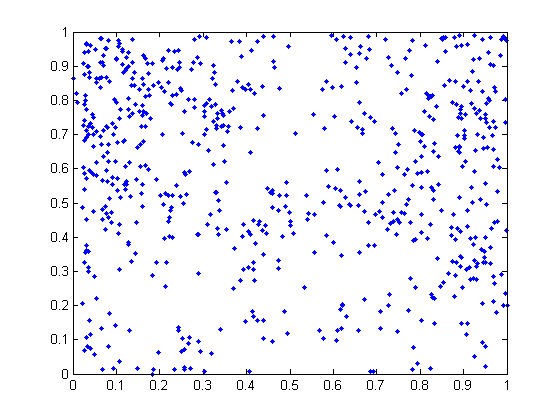Laplace approximation without grid structure

tic
hyp1 = minimize(hyp0, @gp, -100, @infLaplace, @meanConst, cv, lik, X, y);
toc
exp(hyp1.cov)

Function evaluation      0;  Value 1.114380e+03Function evaluation      5;  Value 1.104834e+03Function evaluation      8;  Value 1.098415e+03Function evaluation     12;  Value 1.097589e+03Function evaluation     16;  Value 1.097445e+03Function evaluation     18;  Value 1.097417e+03Function evaluation     23;  Value 1.096718e+03Function evaluation     26;  Value 1.096703e+03Function evaluation     30;  Value 1.096424e+03Function evaluation     33;  Value 1.096419e+03Function evaluation     35;  Value 1.096419e+03Function evaluation     37;  Value 1.096419e+03Function evaluation     46;  Value 1.096419e+03
Elapsed time is 300.589011 seconds.

ans =

0.0815    0.6522    0.0878



Laplace approximation with grid structure and Kronecker inference
tic
hyp2 = minimize(hyp0, @gp, -100, @infGrid_Laplace, @meanConst, cvGrid, lik, Idx, y);
toc
exp(hyp2.cov)

Function evaluation      0;  Value 1.151583e+03Function evaluation     12;  Value 1.124633e+03Function evaluation     14;  Value 1.116309e+03Function evaluation     17;  Value 1.115676e+03Function evaluation     20;  Value 1.115373e+03Function evaluation     23;  Value 1.115308e+03
Elapsed time is 41.778548 seconds.

ans =

0.2346    0.5824    0.1100



Model comparison

The models learned slightly different hyperparameters. Are the likelihoods different?

fprintf('Log-likelihood learned w/out Kronecker: %.04f\n', gp(hyp1, @infLaplace, @meanConst, cv, lik, X, y));
fprintf('Log-likelihood learned with Kronecker: %.04f\n', gp(hyp2, @infLaplace, @meanConst, cv, lik, X, y));

% and what about the likelihood we actually calculated in infGrid_Laplace,
% using the Fiedler bound?
fprintf('Log-likelihood with Kronecker + Fiedler bound: %.04f\n', gp(hyp2, @infGrid_Laplace, @meanConst, cvGrid, lik, Idx, y));

% let's compare our posterior predictions--to save time, we'll use infGrid_Laplace to
% make predictions in both cases (this won't hurt the accuracy, as we explain in the paper.)
[Ef1 Varf1 fmu1 fs1 ll1] = gp(hyp1, @infGrid_Laplace, @meanConst, cvGrid, lik, Idx, y, Idx, y);
[Ef2 Varf2 fmu2 fs2 ll2] = gp(hyp2, @infGrid_Laplace, @meanConst, cvGrid, lik, Idx, y, Idx, y);

figure(1); imagesc([0 1], [0 1], (reshape(Ef1,32,32)')); set(gca,'YDir','normal'); colorbar;
figure(2); imagesc([0 1], [0 1], (reshape(Ef2,32,32)')); set(gca,'YDir','normal'); colorbar;

Log-likelihood learned w/out Kronecker: 1096.4188
Log-likelihood learned with Kronecker: 1100.3819
Log-likelihood with Kronecker + Fiedler bound: 1115.3084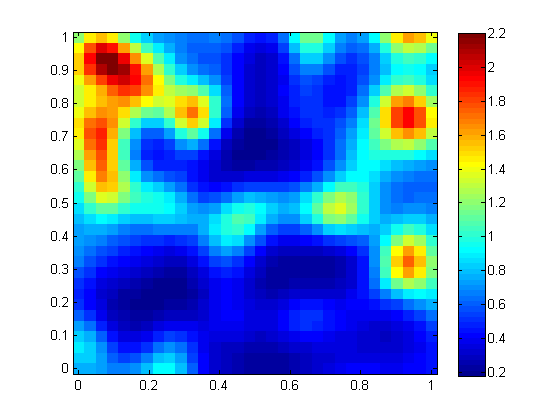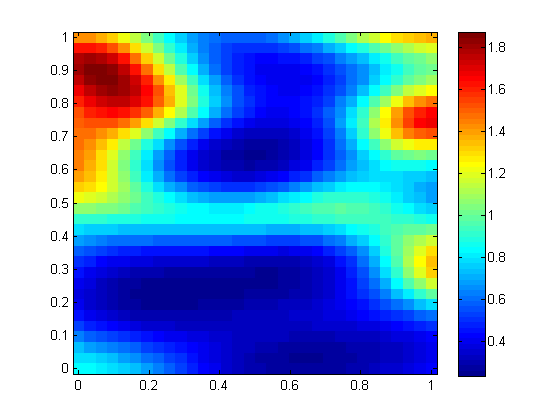Kronecker + grid enables a finer resolution

now let's exploit the grid structure to get a finer resolution map we'll also switch covariances, just for fun.

hyp.cov = log([.3  1 .2 1]);
lik = {@likPoisson, 'exp'}; hyp.lik = [];
mean = {@meanConst}; hyp.mean = .5;

n1 = 64; n2 = 64;
x1 = linspace(0,1.0001,n1+1); x2 = linspace(-0.0001,1,n2+1);
xg = {(x1(1:n1)+x1(2:n1+1))'/2, (x2(1:n2)+x2(2:n2+1))'/2};

% binning y = histcn(xy,x1,x2);
i = ceil((xy(:,1)-x1(1))/(x1(2)-x1(1)));
j = ceil((xy(:,2)-x2(1))/(x2(2)-x2(1)));
counts = full(sparse(i,j,1));
y = counts(:);
xi = (1:numel(y))';
cov = {@covGrid, { {@covMaterniso,5},  {@covMaterniso,5}}, xg};

opt.cg_maxit = 400; opt.cg_tol = 1e-2;
inf_method = @(varargin) infGrid_Laplace(varargin{:},opt);

tic
hyp2 = minimize(hyp, @gp, -100, inf_method, mean, cov, lik, xi, y(:));
toc
exp(hyp2.cov)

% let's compare our posterior predictions
% since we don't care about variance, we can save memory by doing this:
[post nlZ dnlZ] = infGrid_Laplace(hyp2, mean, cov, lik, xi, y(:));
post.L = @(x) 0*x;
ymu = gp(hyp2, @infGrid_Laplace, mean, cov, lik, xi, post, xi);
figure(3), imagesc([0 1], [0 1], reshape(ymu,size(counts))'),
hold on;
set(gca,'YDir','normal');
plot(xy(:,1),xy(:,2),'w.');
colorbar
hold off

Function evaluation      0;  Value 1.954834e+03Function evaluation      6;  Value 1.946841e+03Function evaluation      8;  Value 1.940877e+03Function evaluation     11;  Value 1.940012e+03Function evaluation     14;  Value 1.938218e+03Function evaluation     17;  Value 1.936884e+03Function evaluation     20;  Value 1.934900e+03Function evaluation     22;  Value 1.933195e+03
Elapsed time is 187.843026 seconds.

ans =

0.3051    0.7512    0.1636    0.7512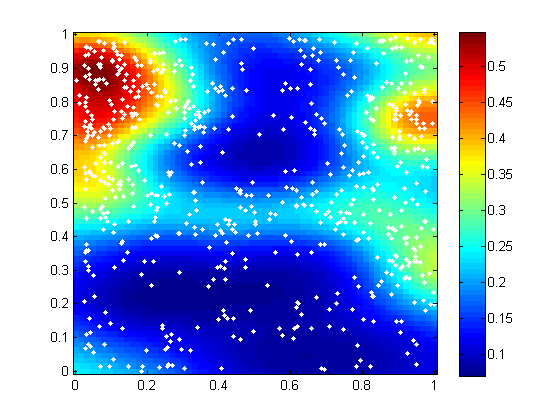Published with MATLAB® R2013a

Kernel Interpolation for Scalable Structured Gaussian Processes (KISS-GP)

Structure exploiting (such a Kronecker and Toeplitz methods) approaches are extremely scalable and exact, but require grid structure.  In previous sections we have extended these approaches to account for partial grids, such as images with missing regions, or spatiotemporal statistics problems with some non-grid data due to lakes, political boundaries, etc.  However, data are often arbitrarily indexed, without any type of grid structure, and thus these structure exploiting methods are not generally applicable.

Conversely, inducing point methods, are popular for their general purpose "out of the box" applicability, without requiring any special structure in the data. However, these methods are limited by requiring a small (m << n) number of inducing inputs, which can cause a deterioration in predictive performance, and the inability to perform expressive kernel learning.

With KISS-GP, we combine the scalability and accuracy of structure exploiting methods with the general applicability of inducing point approaches. These approaches are O(n + g(m)) for inference and learning, and O(1) per test prediction, where m is the number of inducing points, and g(m) ~ m.  The ability to use a large number of inducing points m with little computational expense, enables near-exact GP inference, kernel learning, and predictions on large (up to billions) of datapoints.

The basic idea behind KISS-GP is to create a multidimensional grid of latent inducing points, and exploit the resulting Kronecker, Toeplitz, and Circulant structure in the resulting covariance matrix evaluated over all pairs of these inducing points.  We then perform local kernel interpolation to go back into the observed input space, which may have arbitrary structure.  We can view this approach as creating an approximate kernel given user specified kernel which admits fast numerical linear algebra operations, for inference and learning with Gaussian processes.  The quality of this approximation is often close to exact within numerical precision, given the ability of KISS-GP to use many inducing points.

In short, given a user-specified kernel k(x,z) we introduce an approximate kernel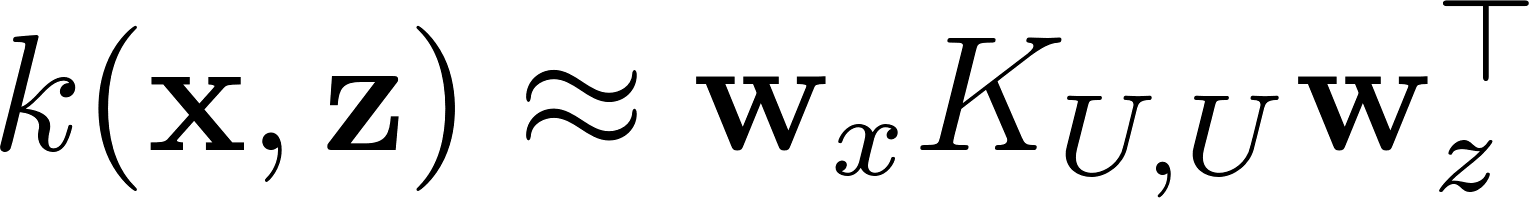where K_{U,U} is evaluated on a set of m virtual inducing points U, located to create structure which will allow K_{U,U} to decompose as a Kronecker product of Toeplitz matrices.  w_x and w_z are sparse interpolation vectors.  This approximate kernel admits fast numerical linear algebra operations for inference and learning with Gaussian processes.

The full details are described in these

Main references:

Kernel interpolation for scalable structured Gaussian processes (KISS-GP)
Andrew Gordon Wilson and Hannes Nickisch
International Conference on Machine Learning (ICML), 2015
Oral Presentation
[PDF, Supplement, arXiv, BibTeX, Theme Song, Video Lecture]

Thoughts on Massively Scalable Gaussian Processes
Andrew Gordon Wilson, Christoph Dann, and Hannes Nickisch
arXiv pre-print, 2015
(See KISS-GP and Deep Kernel Learning for more empirical demonstrations).
[arXiv, PDF, BibTeX, Music]

Video Lecture: KISS-GP Introduction at ICML 2015

Tutorial:

Note: KISS-GP has been added to the new GPML v4.0!  To see a new demo of KISS-GP in the new GPML, demonstrating much of the functionality, download GPML v4.0 and follow demoGrid1d.m and demogrid2d.mFor more experience and understanding of KISS-GP, you can follow the tutorial below, which relies on an older version of GPML that uses a different interface.  Make sure that the correct files are in your Matlab path for each demo.

The key files are covGrid.m and infGrid.m
Read the documentation in these files closely: it will tell you how to
switch on/off Toeplitz structure, Circulant structure, BTTB structure, Kronecker structure, etc.,
and how to switch interpolation strategies.

Step 3: Run all the files in /test
Step 4: Run the the files in /examples.  In
tea_hn_agw.m, vary:

n
: the number of training points
ng: the number of grid (inducing) points
ns: the number of test points

In constructing the grid, pay close attention to whether single braces { or double braces {{ are used.
Double braces enables the BTTB functionality.

Step 5: Run the supervised projection example in experiment_2d.m
Pay close attention to the helper function covGrid('create',...) in both
covGrid.m, but also in how it is called in proj_gauss.m.

This helper function is extremely valuable for creating grids of inducing points based on standard input data x in n x D format, where n is the number of input points, and D is the number of input dimensions.  This helper function can specify whether we want a uniform grid, how many grid points we want, the boundaries of the grid, and whether we use, for instance, k-means, to find locations for the grid points (in this case we could just exploit Kronecker structure but not Toeplitz structure).

For any problem with greater than 1D inputs, I would highly recommend using covGrid('create'), and getting practice with its use.

One of the defining features of KISS-GP is performing local kernel interpolation.  You can see the routines for local kernel interpolation, including multidimensional interpolation, in covGrid.m.  However, to get an intuition of how we use local kernel interpolation to create a fast approximate kernel, given a user specified kernel, take a look at the stripped down 1D demo fitc_grid_poly.m, with associated interpolation script intermat.m.

For higher dimensional inputs, and KISS-GP with kernel learning, see Deep Kernel Learning. This is the code I would recommend using for good performance on multidimensional input problems, with a reasonable number (e.g. n > 1000) datapoints.

Deep Kernel Learning

KISS-GP allows us to naturally create and exploit algebraic structure for highly scalable inference and learning, without requiring input data on a grid.  However, to exploit Kronecker structure, the inducing points in KISS-GP must live in a low dimensional space (e.g. D<5).  The paper "Thoughts on massively scalable Gaussian processes" discusses how to learn supervised projections of the data inputs into this low dimensional, focusing on linear projections.

We can also use very flexible non-linear projections of the kernel inputs, such as deep feedforward or convolutional networks.  Note that in regression, for example, deep feed-forward neural networks will nearly always project into a one dimensional output space.

By learning a deep transformation of the kernel inputs, we are both learning a very flexible (and also interpretable) deep kernel, with all the structural properties and inductive biases of deep learning models, while retaining the non-parametric flexibility and probabilistic representation of a Gaussian process.  Moreover, these supervised deep projections bring us into a low dimensional space where we can apply the scalability ideas of KISS-GP, for O(n) inference and O(1) predictions.

We test deep kernel learning on a wide range of regression problems (the entire UCI repository), with a variety of different input dimensionalities, data sizes, and properties, showing the general applicability of this approach.  We also combine deep kernel learning with spectral mixture kernels

The basic idea is to start with a base kernel, with parameters \theta, using a non-linear function g with its own parameters w.
We take g to be given by a deep architecture.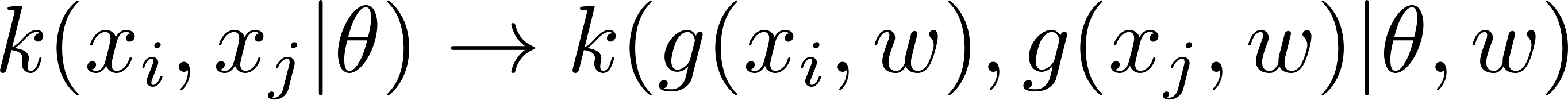We then replace the exact deep kernel with the approximate KISS-GP approximation for this kernel.  We then learn both the parameters theta and w using the marginal likelihood of the Gaussian process.

We often find using spectral mixture base kernels provides a good boost in performance.

Main references:

Deep Kernel Learning
Andrew Gordon Wilson*, Zhiting Hu*, Ruslan Salakhutdinov, and Eric P. Xing
Artificial Intelligence and Statistics (AISTATS), 2016
[PDF, arXiv, BibTeX]

Thoughts on Massively Scalable Gaussian Processes
Andrew Gordon Wilson, Christoph Dann, and Hannes Nickisch
arXiv pre-print, 2015
(See KISS-GP and Deep Kernel Learning for more empirical demonstrations).
[arXiv, PDF, BibTeX, Music]

Here we include the code to implement Deep Kernel Learning
, and to reproduce many of the results in the paper.  It requires GPML 3.6 and Caffe as dependencies.   The GPML version used is included in the zip.  Note that this code is based on an older version of covGrid and infGrid for KISS-GP, which includes flags for circulant embeddings.
You will need to use these versions in your path instead of the newer versions for the deep kernel learning code to run.  Please also pay close attention to how the spectral mixture base kernels are initialized: initialization is fundamental to good performance for these base kernels.

We provide 3 (examples/) :

• UCI Kin40K regression (section 5.1 of the ppaer)
• MNIST digit magnitude extraction (section 5.3)
• Olivetti face orientation extraction (section 5.2)

## Installation

The modified caffe library is under caffe/. Please refer to the installation instructions for prerequisites and configurations. Run the following commands for installation:

make
make matcaffe


## DKL Example: Olivetti Face

We take the olivetti face task for example.

### Preparing data

cd caffe
unzip examples/face/data/face_images.zip
sh examples/face/create_face.sh  # create databases for caffe CNN training and DKL training


Databases will be created under caffe/examples/face/data

### Pre-training CNN

Under caffe/:

sh examples/face/train_face.sh


Please refer to the tutorial for CNN configurations, etc.

After training you will see the trained CNN model caffe/examples/face/face_iter_6000.caffemodel. We have also provided a pre-trained model caffe/examples/face/face_iter_6000.caffemodel.example, so that you can skip this step and go straight to DKL model training.

### Training DKL models

The DKL codes are under examples/face, where dkl_rbf_face.m uses the RBF kernel as the base kernel, and dkl_sm_face.muses the spectral mixture kernel as the base kernel. Training parameters are configured at the beginning of the respective files, including the pre-trained CNN model for initialization, number of training iterations, learning rate, and grid size, etc.

Under examples/face, run the models using matlab

run ../../gpml/startup.m  % setup gpml
run dkl_rbf_face.m  % or dkl_sm_face.m


### Notes

• For preparing the MNIST dataset, run
cd caffe
sh examples/mnist/data/get_mnist.sh
sh examples/mnist/create_mnist.sh

• For preparing the Kin40k dataset, simply run sh examples/kin40k/create_kin40k.sh under caffe/
• Use GPU for the DNN part to speedup training

Stochastic Variational Deep Kernel Learning

We may want to use Gaussian processes and KISS-GP with stochastic gradient descent and mini-batches, for further scalability. However, the Gaussian process marginal likelihood does not factorize.  Here we introduce stochastic variational deep kernel learning, which allows for (1) stochastic gradient mini-batch training in combination with KISS-GP scalability, and (2) deep kernel learning on non-Gaussian likelihood problems and classification.  We apply this approach to a variety of benchmark classification problems, including a large airline passenger dataset, CIFAR and ImageNet.

Main reference:

Stochastic Variational Deep Kernel Learning
Andrew Gordon Wilson*, Zhiting Hu*, Ruslan Salakhutdinov, Eric P. Xing
To appear in Neural Information Processing Systems (NIPS), 2016
[arXiv, PDF]

Here we provide code to reproduce results in the paper.  We focus on classification problems.

The GP-LSTM

Many applications in speech, robotics, finance, and biology deal with sequential data, where ordering matters and recurrent structures are common. However, this structure cannot be easily captured by standard kernel functions. To model such structure, we propose expressive closed-form kernel functions for Gaussian processes. The resulting model, GP-LSTM, fully encapsulates the inductive biases of long short-term memory (LSTM) recurrent networks, while retaining the non-parametric probabilistic advantages of Gaussian processes. We learn the properties of the proposed kernels by optimizing the Gaussian process marginal likelihood using a new provably convergent semi-stochastic procedure and exploit the structure of these kernels for fast and scalable training and prediction. We demonstrate state-of-the-art performance on several benchmarks, and investigate a consequential autonomous driving application, where the predictive uncertainties provided by GP-LSTM are uniquely valuable.

For scalability, we introduce a new provably convergent semi-stochastic training procedure for kernel learning via GP marginal likelihoods, and combine this procedure with KISS-GP.

Learning Scalable Deep Kernels with Recurrent Structure
Maruan Al-Shedivat, Andrew Gordon Wilson, Yunus Saatchi, Zhiting Hu, Eric P. Xing
arXiv pre-print

The codebase contains tutorial examples.  It also provides an alternative implementation of Deep Kernel Learning using Keras with Theano or Tensorflow, and shows how to call KISS-GP functionality from Python.  However, it is still in alpha testing, and may not be as stable as the Caffe based Deep Kernel Learning code.

Datasets

In several papers, we have compared against all UCI regression datasets:

À la carte - learning fast kernels
Zichao Yang, Alexander J. Smola, Le Song, and Andrew Gordon Wilson
Artificial Intelligence and Statistics (AISTATS), 2015
Oral Presentation
[PDF, BibTeX]

Deep Kernel Learning
Andrew Gordon Wilson*, Zhiting Hu*, Ruslan Salakhutdinov, and Eric P. Xing
Artificial Intelligence and Statistics (AISTATS), 2016
[PDF, arXiv, BibTeX]

We provide these data here (kindly compiled by Zichao Yang) with the normalization and pre-processing that we used, so that you have a way to test whether this code is working for you: can you reproduce the results in Table 1 of Deep Kernel Learning?
Be sure to run the included pre-processing scripts on the raw data and verify some of the results!

These data also provide an easy way to benchmark your own methods on all of these problems.  If you use the data for this purpose, please provide a reference to this webpage.  Since the code for many of the methods we used are available (e.g. DKL), please verify some of these results using the code here before taking the results in the table for granted in your own comparison.  You may, for instance, have forgotten to run the pre-processing or normalization routines, or the pre-processing may have changed between posting these data and the writing of those papers.  You may also not be applying the code on this page correctly on other problems, so testing on these data provides a sanity check.

Many thanks to my wonderful collaborators.  Particular thanks to Hannes Nickisch, Zhiting Hu, and Maruan Al-Shedivat, for their contributions to the implementations of KISS-GP, Deep Kernel Learning, and the GP-LSTM, respectively.